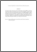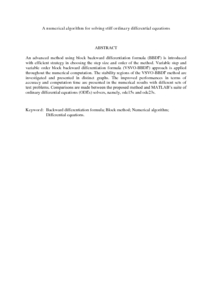# A numerical algorithm for solving stiff ordinary differential equations

## Citation

Mohd Yatim, Siti Ainor and Ibrahim, Zarina Bibi and Othman, Khairil Iskandar and Suleiman, Mohamed (2013) A numerical algorithm for solving stiff ordinary differential equations. Mathematical Problems in Engineering, 2013. art. no. 989381. pp. 1-11. ISSN 1024-123X

## Abstract

An advanced method using block backward differentiation formula (BBDF) is introduced with efficient strategy in choosing the step size and order of the method. Variable step and variable order block backward differentiation formula (VSVO-BBDF) approach is applied throughout the numerical computation. The stability regions of the VSVO-BBDF method are investigated and presented in distinct graphs. The improved performances in terms of accuracy and computation time are presented in the numerical results with different sets of test problems. Comparisons are made between the proposed method and MATLAB’s suite of ordinary differential equations (ODEs) solvers, namely, ode15s and ode23s.Preview
PDF (Abstract)
A numerical algorithm for solving stiff ordinary differential equations.pdfView Item